Math resources Geometry Triangles

Scalene triangle

# Scalene triangle

Here you will learn about scalene triangles, including what a scalene triangle is and the properties of scalene triangles.

Students first learn about triangles in kindergarten and 1st grade in geometry with their work in reason with shapes and their attributes. They expand their knowledge of triangles as they progress through elementary school and into secondary school.

## What is a scalene triangle?

A scalene triangle is a type of triangle that has no equal side lengths and no equal angle measurements, which means the sides and angles are not congruent.

Properties of a scalene triangle:

• No equal sides.
• No equal angles.

The dash marks on the sides and the angles do not match up. This shows that the triangle does not have congruent sides and does not have congruent angles.

There are three types of scalene triangles:

Obtuse scalene triangleAcute scalene triangleRight scalene triangle

● No equal sides
1 obtuse angle

● No equal sides
3 acute angles

● No equal sides
1 right angle ( 90^{\circ})

### Symmetry

A scalene triangle has no lines of symmetry.

Notice how none of the lines of symmetry cut the scalene triangle into two equal halves that are reflections of each other.

### What is a scalene triangle?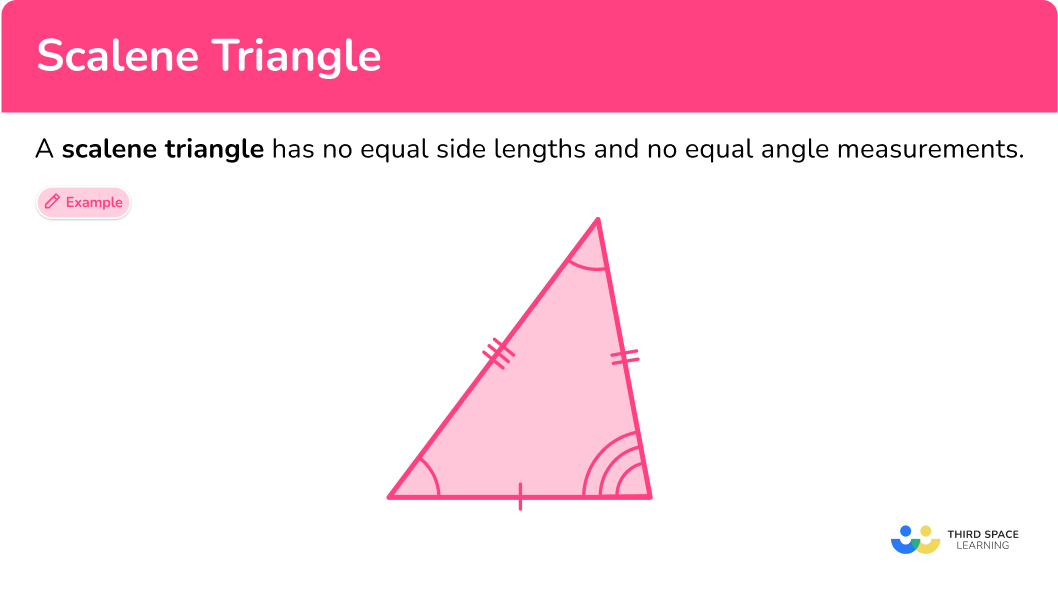## Common Core State Standards

• Grade 4 – Geometry (4.G.A.3)
Recognize a line of symmetry for a two-dimensional figure as a line across the figure such that the figure can be folded along the line into matching parts. Identify line-symmetric figures and draw lines of symmetry.

• Grade 5 – Geometry (5.G.B.3)
Understand that attributes belonging to a category of two-dimensional figures also belong to all subcategories of that category.

## How to identify a scalene triangle

In order to identify scalene triangles:

1. Recall the definition.
2. Explain why the triangle fits or does not fit the definition.

## Scalene triangle examples

### Example 1: classify scalene triangle

Select the triangle that is scalene.

1. Recall the definition.

A scalene triangle is a triangle that has no equal sides and no equal angles.

2Explain why the triangle fits or does not fit the definition.

Triangle A is not the correct choice because it has two equal (congruent) sides, so it is an isosceles triangle.

Triangle C is not the correct choice because it has two equal (congruent) sides, so it is also an isosceles triangle.

Triangle B is the correct choice because none of the sides are equal.

### Example 2: classify scalene triangle

Is the triangle below a scalene triangle?

A scalene triangle is a triangle that has no equal sides and no equal angles.

The triangle has three different side lengths: 9 \, cm, \, 19 \, cm, and 23 \, cm. So, the triangle is a scalene triangle.

### Example 3: classify scalene triangle

How many lines of symmetry does the scalene triangle have?

A line of symmetry divides a shape into two equal parts that are reflections or mirror images of each other.

There is no way to draw a line through the triangle that divides it into two equal parts. Scalene triangles do not have any lines of symmetry because they do not have any equal sides or angles.

### Example 4: classify scalene triangle

What type of scalene triangle is pictured below?

An obtuse scalene triangle is a scalene triangle with 1 obtuse angle.

An acute scalene triangle is a scalene triangle with 3 acute angles.

A right scalene triangle is a scalene triangle with 1 right angle.

The triangle in the picture has the angle measurements 35^{\circ}, \, 70^{\circ}, \, 75^{\circ}, and each of the angle measures are acute angles because they are greater than 0^{\circ} and less than 90^{\circ}.

The triangle is an acute scalene triangle.

### Example 5: classify scalene triangle

What type of scalene triangle is pictured below?

An obtuse scalene triangle is a scalene triangle with 1 obtuse angle.

An acute scalene triangle is a scalene triangle with 3 acute angles.

A right scalene triangle is a scalene triangle with 1 right angle.

The triangle pictured has the angle measurements 20^{\circ}, \, 35^{\circ}, \, 125^{\circ}, and one of the angle measurements is an obtuse angle because it is greater than 90^{\circ} and less than 180^{\circ}.

So the triangle is an obtuse scalene triangle.

### Example 6: classify scalene triangle

In math class, Mikey and Nicole’s teacher asked the students to identify what type of triangle has side lengths 5 \, cm, \, 7 \, cm, and 8 \, cm.

Mikey says the triangle is a right triangle and Nicole says the triangle is a scalene triangle. Who is correct?

A right triangle is a triangle with one right angle.

A scalene triangle is a triangle with no equal side lengths.

Since the teacher did not give any specific information about the angles of this triangle, you can only classify the triangle by its sides. The side measurements are all different: 5 , cm, \, 7 \, cm, and 8 \, cm.

So, Nicole is correct. The better classification of this triangle is that it is scalene.

### Teaching tips for scalene triangles

• Incorporate drawing activities where students can use rulers and protractors to create different types of scalene triangles and compare and contrast them to other types of triangles.

• Incorporate origami activities that are creative and help students understand the properties of triangles.

• Although worksheets have their place for practicing skills, using digital platforms for game playing is fun for students and helps them to practice skills. Check out free platforms such as desmos, quizlet, blooket, and quizziz.

### Easy mistakes to make

• Not taking notice of both side and angle relationships when classifying triangles
Be sure to notice the side relationships and the angle relationships of triangles before classifying them. It could lead to confusion if you only use the sides or only the angles.

• Mixing up the names of triangles
A question may ask a student to explain the difference between an isosceles triangle and a scalene triangle. Knowing the vocabulary helps students with questions like these.

### Practice scalene triangle questions

1. Which of these triangles is a scalene triangle?A scalene triangle has no equal sides and no equal angles. This was the only triangle that did not have any markings on the sides and angles as being equal (congruent).The other triangles are marked with equal sides and equal angles, so they are not scalene triangles.

2. How many lines of symmetry does a scalene triangle have?

1302As a scalene triangle has no equal sides or equal angles, it has no lines of symmetry.

In order for a triangle to have symmetry, it would need to have at least two equal sides.

3. Select the triangle that is a right scalene triangle.A right scalene triangle is a scalene triangle (no equal sides) with 1 right angle.

This triangle was the only triangle with 1 right angle and three sides that were not equal.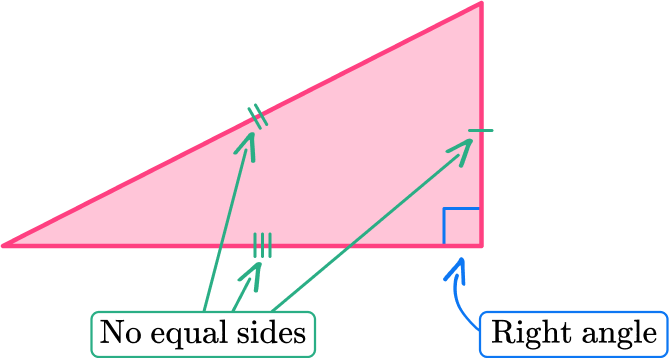4. Select the triangle that is an obtuse scalene triangle.An obtuse scalene triangle is a scalene triangle (no equal sides) with 1 obtuse angle.

This triangle was the only triangle with one angle greater than 90^{\circ} and less than 180^{\circ} and three unequal sides.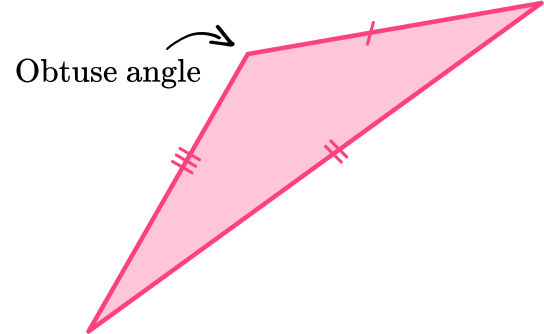5. The angles of a triangle are 57^{\circ}, \, 58^{\circ}, and 65^{\circ}. What type of triangle is this?

Obtuse isosceles triangleAcute isosceles triangleAcute scalene triangleObtuse scalene triangleAn acute scalene triangle is a triangle with three angles that are acute. The triangle also has three sides of different lengths and three angles with different measures.

So a triangle with angle measurements, 57^{\circ}, \, 58^{\circ}, and 65^{\circ} is an acute scalene triangle because all the angle measurements are greater than 0^{\circ} and less than 90^{\circ}.

6. Which photo represents a right scalene triangle?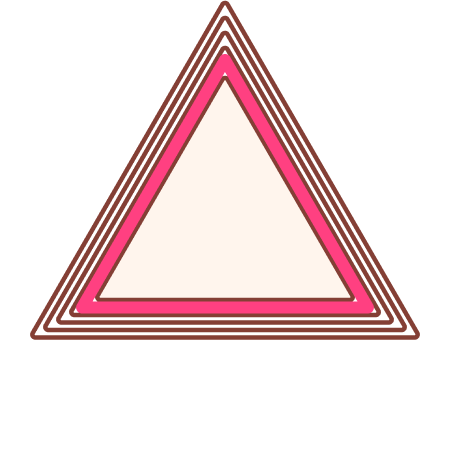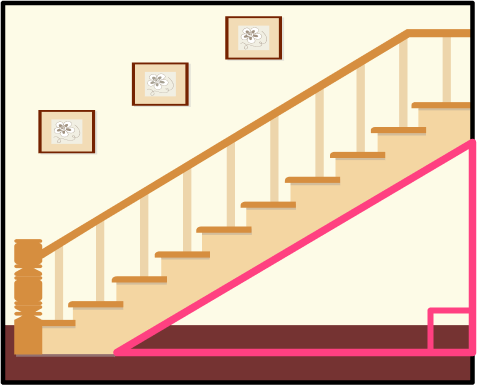A right scalene triangle is a scalene triangle (no equal sides and no equal angles) with 1 angle being 90^{\circ}.

This photo was the only picture that had a scalene triangle with a right angle.## Scalene triangle FAQs

Can you apply the Pythagorean theorem to scalene triangles?

You can apply the Pythagorean theorem to only right scalene triangles because the Pythagorean theorem only works with right triangles.

How can you find the area of a scalene triangle?

You can find the area of scalene triangles the same way you find the area of other triangles. Use the formula for the area of the triangle, A=\frac{1}{2} \cdot b \cdot h where h represents the height of the triangle or the altitude of the triangle and b represents the base of the triangle.

How can you find the perimeter of a scalene triangle?

You can find the perimeter of scalene triangles the same way you find the perimeter of other triangles, add up the sides.

Can you apply trigonometry ratios to scalene triangles?

Yes, you can apply the trigonometric ratios (sine, cosine, tangent) to any right triangle. You will learn about trigonometry in high school.

What is the semi-perimeter?

The semi-perimeter is half the sum of all of the sides of a triangle. So to find the semi-perimeter of a scalene triangle, sum the sides of a scalene triangle and then take half of that sum.

Are the properties of triangles important to remember in middle school and high school?

Yes, triangles have important properties that you will explore in a deeper context when you take geometry in middle school and high school.

What is heron’s formula?

Heron’s formula is used to find the area of a triangle when you only know the lengths of the sides of the triangle.

## Still stuck?

At Third Space Learning, we specialize in helping teachers and school leaders to provide personalized math support for more of their students through high-quality, online one-on-one math tutoring delivered by subject experts.

Each week, our tutors support thousands of students who are at risk of not meeting their grade-level expectations, and help accelerate their progress and boost their confidence.